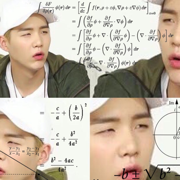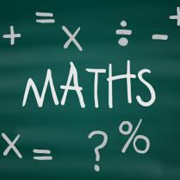# Learn Equations Maths

Study Equations Maths using smart web & mobile flashcards created by top students, teachers, and professors. Prep for a quiz or learn for fun!

### Top Equations Maths Flashcards Ranked by Quality

• Maths EquationsShow Class
• Maths equationsShow Class
• Poker Maths, Equity, pot odds, CombosShow Class
• pure math equationsShow Class
• Maths Gcse EquationsShow Class
• Unity Math 3 Ch3 Equations in 2 VariablesShow Class
• Maths EquationsShow Class
• Maths EquationsShow Class
• Math EquationsShow Class
• maths equationsShow Class
• Maths EquationsShow Class
• Aviation Math EquationsShow Class
• Maths Equations to learnShow Class
• SMC MATH 326 Definitions And EquationsShow Class
• A Level Math: Sequences and SeriesShow Class
• A Level Maths equations to memoriseShow Class
• Unity Math 3 Ch2 Equations in 1 VariableShow Class
• Key Equations for Physics and MathsShow Class
• Some Maths EquationsShow Class
• Maths EquationsShow Class
• Maths - Equations(Sin,Cos,Tan)Show Class
• SAT & subject testsShow Class
• ALTIUSShow Class
• GRE PrepShow Class
• ALTIUS CardsShow Class
• Maths!Show Class
• SAT Math EssentialsShow Class
• Ma110Show Class
• Year 10 revisionShow Class
• MathsShow Class
• Maths GCSE 1-9 (Foundation)Show Class
• MCAT chemShow Class
• BusinessShow Class
• MCAT RetakeShow Class
• BCPS ACCP 2017 PharmacotherapyShow Class
• Geek Club: ExtensionShow Class
• MCAT Physics and MathShow Class
• PhysicsShow Class
• SQA MathsShow Class
• schoolShow Class
• MathShow Class
• Biology A- LevelShow Class
• GCSE RevisionShow Class
• BMATShow Class
• revisionShow Class
• VocabularyShow Class
• MCAT ALLShow Class
• Ben's deckShow Class
• Science equationsShow Class
• StudyingShow Class